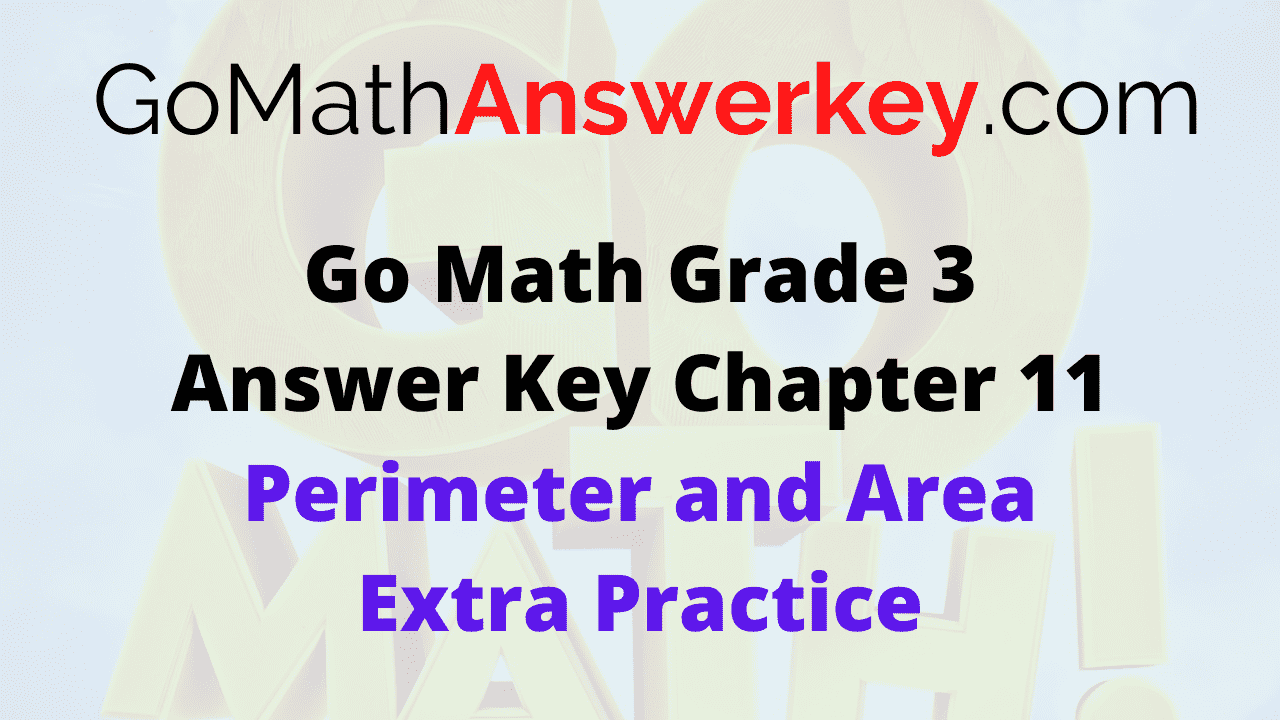# Go Math Grade 3 Answer Key Chapter 11 Perimeter and Area Extra Practice

Go Math Grade 3 Answer Key Chapter 11Perimeter and Area Extra Practice will help to more number of students to get more knowledge on the perimeter and area of the different shapes. By following the Go Math Answer key, students can easily find out the Perimeter and Area of the different shapes like rectangle, square, and etc.. Go Math answer key will give you the perfect answers with a clear explanation to every question in an easy way.

## Go Math Grade 3 Chapter 11 Perimeter and Area Answer Key

Follow the Go Math Grade 3 Chapter 11 Perimeter and Area Answer Key, get the step by step explanation to every question. Each and every student will easily gain the knowledge on Perimeter and Area topic by using the Go Math Grade 3 Chapter 11 Perimeter and Area Answer Key.

### Common Core – Page No. 235000

Chapter 11 Extra Practice

Lessons 11.1, 11.3

Question 1.
Find the perimeter of the shape. Each unit is 1 centimeter.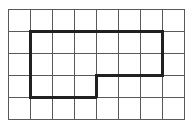______ centimeters

18 centimeters

Explanation: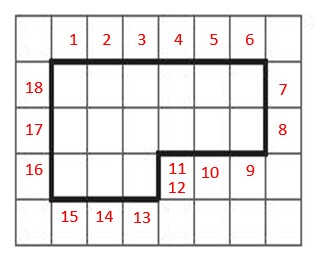Each square in the grid is a 1 by 1-centimeter square. So, we have to do is add up the
lengths of the dark segments right over the figure. Start the count from the box where 1
is placed. This parameter is 1, 2, 3, 4, 5, 6, 7, 8, 9, 10, 11, 12, 13, 14, 15, 16, 17, 18 centimeters long. So, it is 18 centimeters.

Question 2.
The square has a perimeter of 28 inches. What is the length of each side of the square?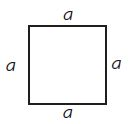______ inches

7 inches

Explanation:
From the given data
Perimeter of a square is = 28 inch
Length of each side of the square is = a inch
Perimeter of a square = a + a + a + a = 4a = 28 inch
4a= 28
Then, a = 28/4= 7
Therefore, length of each side of the square is = a = 7 inch

Lesson 11.2

Use a centimeter ruler to find the perimeter.

Question 3.______ cm

11 cm

Explanation: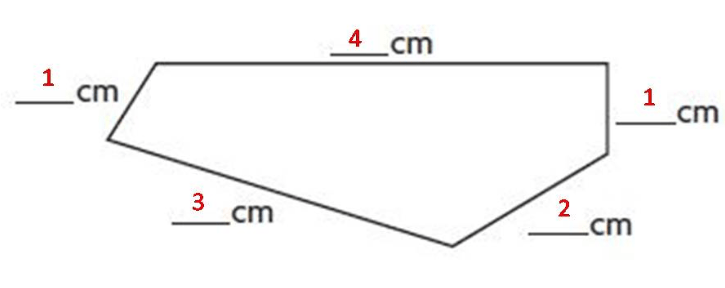1 + 4 + 1 + 2 + 3 = 11 cm

Question 4.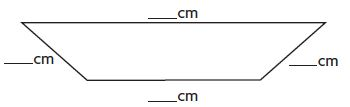______ cm

15 cm

Explanation:5 + 4 + 3 + 3 = 15 cm

Lessons 11.4–11.6

Find the area of the shape.
Each unit square is 1 square inch.

Question 5.Area = ______ square inches

Area = 15 square inches

Explanation:As per the given data,
Each unit square is 1 square inch
Then, the area of the shape is = 15 square inches

Question 6.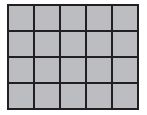Area = ______ square inches

Area = 20 Square inches

Explanation:From the given data,
Each unit square is 1 square inch
Then, the area of the shape = 5 x 4 = 20 Square inches

### Common Core – Page No. 236000

Lesson 11.7

Use the rectangles for 1–2.Question 1.
How do the length and width change from Rectangle A to Rectangle B?
Type below:
__________

22 centimeters

Explanation:
Rectangle A: Length = 2 ft; Width = 2 ft.
Rectangle B: Length = 3 ft; Width = 2 ft.
The Width from Rectangle A to Rectangle B is the same.
The Length is increased 1 ft from Rectangle A to Rectangle B.

Question 2.
How do the areas change from Rectangle A to Rectangle B to Rectangle C?
Rectangle A: ______ sq. ft.
Rectangle B: ______ sq. ft.
Rectangle C: ______ sq. ft.

22 centimeters

Explanation:
Area of Rectangle A: 2 x 2 = 4 sq. ft
Area of Rectangle B: 2 x 3 = 6 sq. ft
Area of Rectangle C: 2 x 4 = 8 sq. ft
Then, areas change from Rectangle A to Rectangle B to Rectangle C is 2 sq. ft

Lesson 11.8

Draw a line to break apart the shape into rectangles.
Find the area of the shape.

Question 3.______ square units

23 square units

Explanation:2 x 7= 14 Sq. units; 3 x 3= 9 Sq. units
14 + 9 = 23 Sq. units
Area of the shape is = 23 Sq. units

Question 4.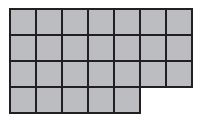______ square units

26 square units

Explanation: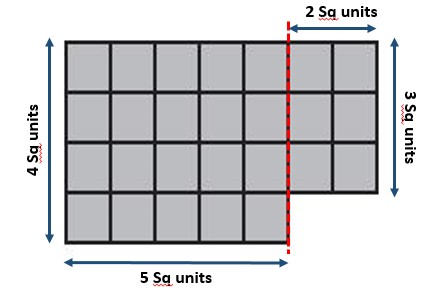4 x 5 = 20 Sq. units; 2 x 3 = 6 Sq. units
20 + 6 = 26 Sq. units
Then, the area of the shape is = 26 Sq. units

Lessons 11.9–11.10

Find the perimeter and area of each rectangle.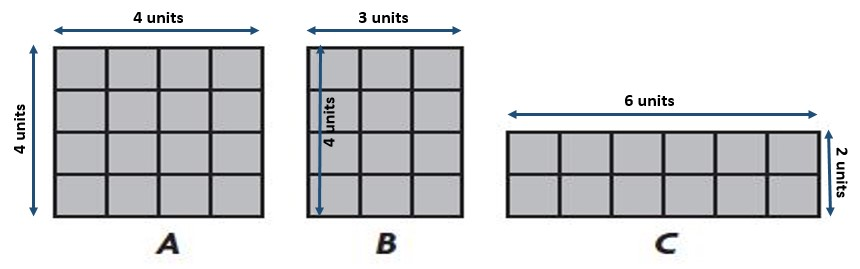Question 5.
Which two rectangles have the same perimeter?
Rectangles ______ and ______

Rectangles A and C

Explanation:
Rectangle A: Permiter = 4 + 4 + 4 + 4 = 16 Units.
Rectangle B: Permiter = 3 + 4 + 3 + 4 = 14 Units.
Rectangle C: Permiter = 6 + 2 + 6 + 2 = 16 Units.
Rectangle A and Rectangle C have the same perimeter

Question 6.
Which two rectangles have the same area?
Rectangles ______ and ______

Rectangles B and C

Explanation:
Rectangle A: Area = 4 x 4 = 16 Sq. Units
Rectangle B: Area = 4 x 3 = 12 Sq. Units
Rectangle C: Area = 6 x 2 = 12 Sq. Units
Rectangle B and Rectangle C have the same area.

### Conclusion

In this Chapter, Go Math explained clearly about Perimeter and Area of different Shapes by using images, indicating images with arrows, and numbers. Every student has a chance to know how to find out the perimeter of shapes and how to find out the area of shapes with the Go Math Grade 3 Perimeter and Area answer key. Get quick knowledge with the Go Math solutions and find out the Perimeter and Area of shapes very quickly.

By following the Go Math Grade 3 solutions, students can quickly find the perimeter and area of shapes within a few minutes. Do not move to anywhere, stay on Go Math, and follow them each and every question of Perimeter and Area with explanation and strengthen your knowledge.

Scroll to Top